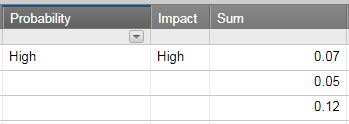#### Welcome to the Smartsheet Forum Archives

The posts in this forum are no longer monitored for accuracy and their content may no longer be current. If there's a discussion here that interests you and you'd like to find (or create) a more current version, please Visit the Current Forums.

# Scoring

Hello,

I am trying to setup a Risk registry where user selects the probability (very high, high, low) and formula in a new column says if High, then score is 0.07.

I then want to do the same thing with Impact (user selects impact high, low etc. A new column puts a score based on formula: if high, then 0.05).

I then need another column that sums the two scores, to provide a risk score.

Have not been able to set this up as the formula's can't be added, it just displays either a - or combines them, such as showing 1+1= 1 1 instead of 2...

Any ideas?

Thanks!

• Hi,

Did you try SUM function? Because "+" is functioning more like CONCATENATE function in Excel.

A1=IF(Probability="High",0.07) (Don't use "" to make sure you get number results.)

A2=IF(Impact="High",0.05) (Don't use "" to make sure you get number results.)

=SUM(A1,A2)This discussion has been closed.